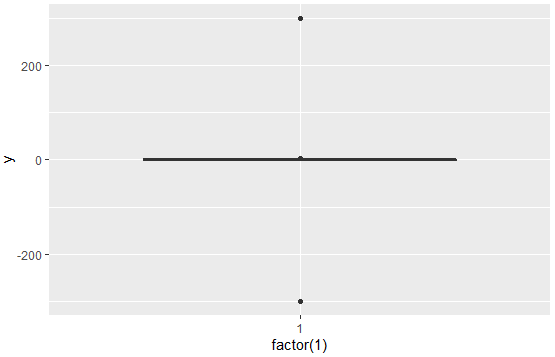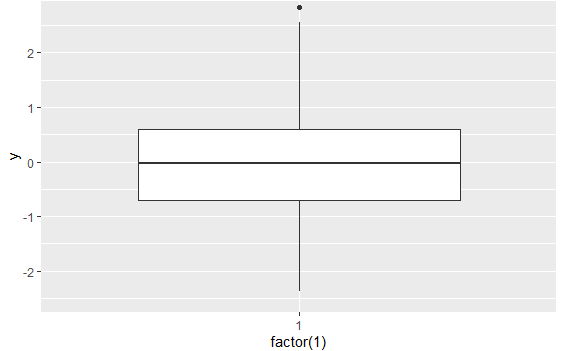2 views

How would I ignore outliers in ggplot2 boxplot? I don't simply want them to disappear (i.e. outlier.size=0), but I want them to be ignored such that the y-axis scales to show 1st/3rd percentile. My outliers are causing the "box" to shrink so small its practically a line. Are there some techniques to deal with this?

Here's an example:

y = c(.01, .02, .03, .04, .05, .06, .07, .08, .09, .5, -.6)

qplot(1, y, geom="boxplot")by

To ignore the outliers, you can use the boxplot.stats function to compute the lower and upper whiskers of the plot and then scale the y-limits accordingly.

For example:

df = data.frame(y = c(-300, rnorm(300), 300))

Plot with outliers:

p = ggplot(df, aes(y = y)) + geom_boxplot(aes(x = factor(1)))Computing whiskers:

ylim1 = boxplot.stats(df\$y)\$stats[c(1, 5)]

Plot without outliers: